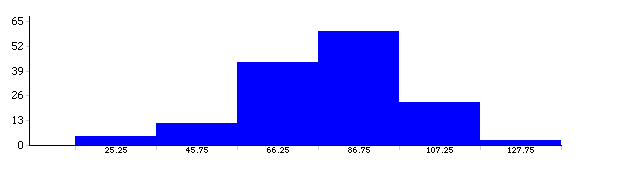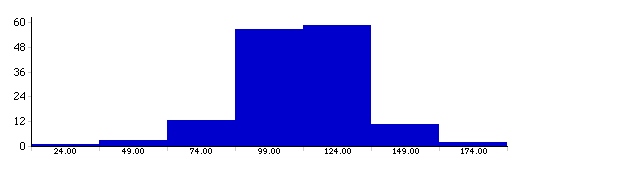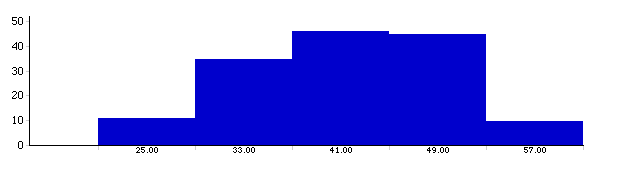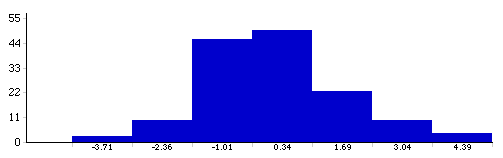Descriptive Statistics

The first step in examining the relationship between strength and job performance is to look at some basic descriptive statistics for each of the four variables. Measures of central tendency such as the mean and the median can be used to determine the location of the distribution of scores. Measures of dispersion such as the range (minimum, maximum), the standard deviation, and the variance tell you how spread out the scores were.

Arm

For the ARM test, we can see that the 147 study participants exerted an average of 78.75 pounds of force. Half of the participants exerted less than 81.50 pounds of force while the other half scored greater than 81.50. The scores were fairly spread out with a standard deviation of 21.11. The lowest score was 19 lbs. Someone managed to exert a maximum score of 132 lbs.

 N Mean Median Trimean Minimum Maximum 25th percentile 75th percentile s se of mean Skew Kurtosis 147 78.75 81.50 80.38 19.00 132.00 64.50 94.00 21.11 1.74 -0.30 0.01

Another important step in evaluating a set of data is to look at the overall shape of the distributions. One way of doing that is to construct a stem-and-leaf graph like the one below.How many people exerted a force of 85 pounds?
2
3
4
5Another good way to portray the shape of the distribution is with a histogram as shown below.Descriptive statistics and histograms for the remaining variables are presented below.

Grip

 N Mean Median Trimean Minimum Maximum 25th percentile 75th percentile s se of mean Skew Kurtosis 147 110.23 111.00 110.12 29.00 189.00 94.00 124.50 23.63 1.95 0.02 1.14

If the distribution were normal, between which two values would 68% of the values lie?
[0,1]
[109,111]
[85, 136]
[90, 131]
[87, 134]Ratings

 N Mean Median Trimean Minimum Maximum 25th percentile 75th percentile s se of mean Skew Kurtosis 147 41.01 41.30 41.30 21.60 57.20 34.80 47.80 8.52 0.70 -0.11 -0.84The ratings variable:
appears to deviate from normality.
appears to approximately normally distributed.SIMS

 N Mean Median Trimean Minimum Maximum 25th percentile 75th percentile s se of mean Skew Kurtosis 147 0.20 0.16 0.11 -4.17 5.17 -0.99 1.11 1.68 0.14 0.45 0.68Notice the distribution has a slight positive skew (skewed to the right).